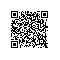# 算法学习之路|二分图的最大匹配—匈牙利算法（Dfs实现）

struct Edge
{
int from；
int to;
};
vector <int> G[max];//存储i点的出发点编号
int matchingnode[max];
int check[max];
int Dfs(int u)
{
vector <int>::iterator it;
for(it=G[u].begin();it!=G[u].end();it++)
{
int v=*it;
if(!check[v])//判断是否在交替路中
{
check[v]=1;
if(matchingnode[v]==-1||Dfs(matchingnode[v]))//是否是匹配点
{
matching[v]=u;
matching[u]=v;
return 1;
}
}
}
return 0;
}
int hungarian ()
{
int _count;
memset(matchingnode,-1,sizeof(matchingnode));
for(int i=0;i<max;i++)
{
if(matchingnode[i]==-1)
{
memset(check,0,sizeof(check));
if(Dfs(i))
_count++;
}
}
return _count;
}使用钉钉扫一扫加入圈子
+ 订阅